• 今天小编就为大家分享一篇python交换两个变量方法，具有很好的参考价值，希望对大家有所帮助。一起跟随小编过来看看吧
• 在考试和面试时经常碰见交换两个变量，下面我就罗列出Python 语言交换两个变量的4种方法 方法1： ""常规方法，采用第三方变量"" x=2 y=3 z= x # z=2 x = y # x = 3 y=z # y =...
在考试和面试时经常碰见交换两个变量的值，下面我就罗列出Python 语言交换两个变量的值的4种方法
方法1：
""常规方法，采用第三方变量""
x=2
y=3
z= x               #  z=2
x = y              #  x = 3
y=z               #  y = 2
fang
方法2：
不使用中间变量
x= x+y    #  x=2+3=5
y=x-y      #  y=5-3=2
x=x-y     #   x= 5-2=3
PS:两个数量相加，可能超出该类型的取值范围
方法3：
x = x^y  # 1
y=x^y    # 2
x=x^y    # 3
此方法效率高，可读性太差
方法4：
a,b=b,a
此方法时python特有的语法，推荐使用


展开全文• method 1： a，b = b，a method 2： a = a+b b = a-b ... 您可能感兴趣的文章:python交换两变量的值方法对Python中实现两个数的值交换的集中方法详解对变量赋值的理解–Pyton中让两个值互换的实现方法
• 方式一：使用第三个变量的常规交换方式 a = 18 b = 30 temp = 0 temp = a a = b b = temp 方式二：python中特有的交换方式 a = 18 b = 30 a, b = b, a 方式三：使用加减法 a = 18 b = 30 a = a + b - a b = b + a ...
方式一：使用第三个变量的常规交换方式
a = 18
b = 30
temp = a
a = b
b = temp

方式二：python中特有的交换方式
a = 18
b = 30
a, b = b, a

方式三：使用加减法
a = 18
b = 30

a = a + b   # a = 48
b = a - b   # b = 18
a = a - b   # a = 30

方式四：使用异或法
python中一个数连续异或另一个数的结果还是这个数
a = 18
b = 30
a =  b ^ a ^ a
b = a ^ b ^ b

展开全文• python交换两个变量值的几种方式 1.python中独有的，也是最简单的方式： a,b=b,a 2.引入第三个变量，可以看作两装满牛奶的瓶子a和瓶子b，如果我想交换这两瓶子中的牛奶，就需要一空瓶temp，先将a瓶中的...
python中交换两个变量值的几种方式
1.python中独有的，也是最简单的方式：
a,b=b,a
2.引入第三个变量，可以看作两个装满牛奶的瓶子a和瓶子b，如果我想交换这两个瓶子中的牛奶，就需要一个空瓶temp，先将a瓶中的牛奶倒入空瓶temp中，此时a瓶为空，可以将b瓶中的牛奶倒入a中，然后再将temp中的牛奶倒入b中，这样就实现了交换。
temp=a
a=b
b=temp
3.采用特殊的运算方式：
a=1
b=2

a=a+b #1+2=3
b=a-b #3-2=1
a=a-b #3-1=2
4.使用python中位运算中异或^进行操作：
a=1 #转换成二进制数为01
b=2 #转换成二进制数为10

a = a ^ b #01^10-->11
b = a ^ b #11^10-->01
a = a ^ b #11^01-->10
异或操作是先将两个数转换成对应的二进制数，然后对两个二进制数进行按位异或，即两个数字不同则为1，否则为0。如1^0=1,0^0=0。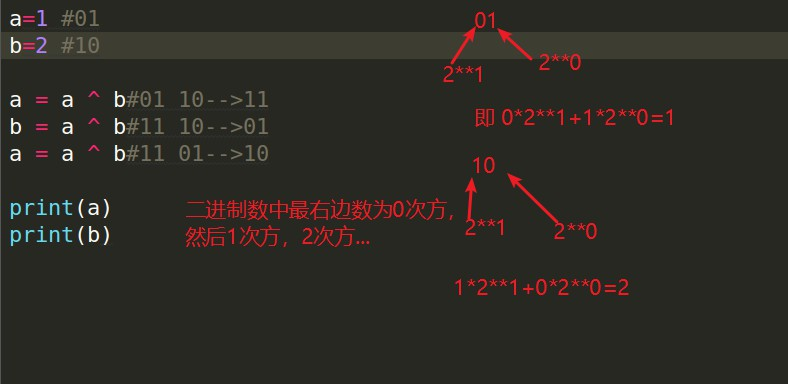展开全文• 交换两个变量方法有很多，但大多数情况下被问到这问题的时候，总是让我们回答不常用的方法，下面我们讨论一下交换变量的几种方法： 方法一： 该方法是我们最常见的方法，也是最容易理解的方法，通过添加新...
交换两个变量的值方法有很多，但大多数情况下被问到这个问题的时候，总是让我们回答不常用的方法，下面我们讨论一下交换变量的几种方法：

方法一：
该方法是我们最常见的方法，也是最容易理解的方法，通过添加新的中间变量的方法实现交换数值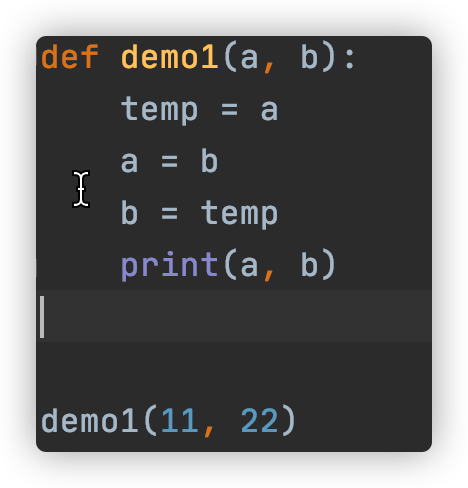方法二：
此方法是python中特有的方法，一行代码就可以解决问题，非常快捷。是将变量放到元组中，再通过元组按照index进行赋值的方式对变量进行重新赋值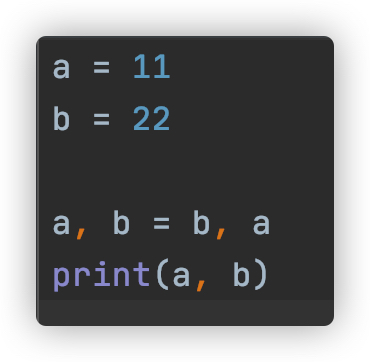当然这种方法并不受限于两个变量，多个变量也是可以的，只是要对应好哪个变量与哪个变量交换值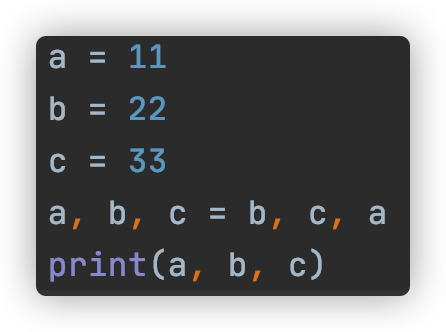方法三：
通过简单的逻辑运算进行两个值的计算，这个方法想到的同学就会少一些了，这种方法只是效率低了点，但仍能实现交换的效果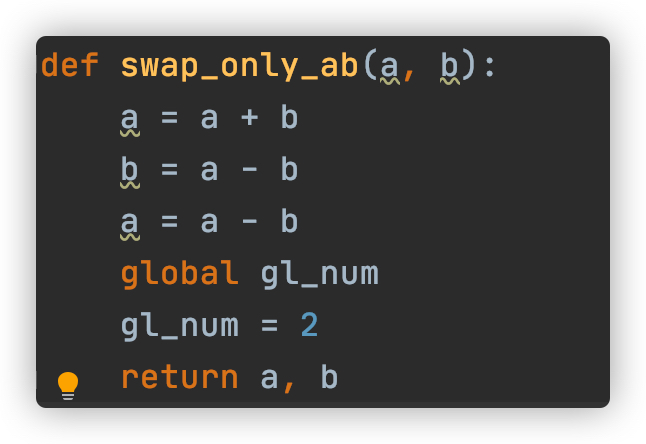方法四：
通过异或运算，将两个值进行互换，能想到这种方法的同学更是少之又少。
异或运算简单点来说就是计算机会先把十进制数转化为二进制数，并且对二进制数进行从右到左进行比较，如果比较的两个二进制数相同，结果为0，不同结果为1，1^1=0，1^0=1，0^0=0。
使用这种方法是不是更加炫酷了？通过按位异或的方式可以实现两个变量值的交换，并且相比于其他方法，可以减少变量的定义以及减少计算机对代码的解析时间。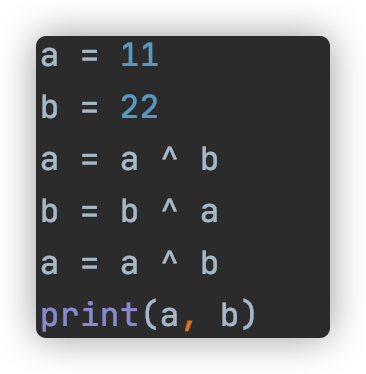这四种方法都可以实现交换两个变量值的要求，如果还有更好的方法或者文章中错误的地方，欢迎各位同学指正交流。


展开全文• 有a,b两个变量，需要交换两个变量： 1.借助中间变量temp temp = a a = b b = temp 2.变量之间进行运算替换 a = a + b b = a - b a = a - b 3.python独有的方法 a, b = b, a 这方法是Python独有，其他语言不...
• Python如何交换两个变量？第一种方法（所有编程语言通用）：第二种方法（适用python）： 第一种方法（所有编程语言通用）： 想要交换两个变量，就要再多定义一个变量。 例如：要交换变量a,b的，就需要再...
• 如果想交换两个变量a,b的，我们有三种解法 第一种 用第三个变量存储 c=a a=b b=c 第二种 加减法 a=a+b b=a-b a=a-b 第二行的a已经不是原来的a了，是a+b，我们第二行表示的b是交换后的a,第三行a是a+b，...
• python中四种交换两个变量的方法 方法一： 通过中间变量的方式，交换数值 def swap(a, b): temp = a a = b b = temp print(a, b) 方法二： python特有方法 直接将a, b两个变量放到元组中，再通过元组...
• 如果你是使用过c语言的朋友，提到交换两个变量中的数值就会想到需要定义一新的变量，就比如需要把连杯子中的水换位置，那么就需要拿来另外一杯子，将其中的一杯子里的水倒进这新拿来的杯子中，然后将...
• 大部分语言，例如c语言，交换两个变量需要使用中间变量。 例如交换a,b 伪代码：tmp = a a = b b = apython里面可以实现无临时变量的交换 （a,b) = (b,a) 对于它的交换原理我深感好奇，因为这意味着python...
• python交换两个变量为什么不用中间变量，编译器在中间都做了什么？是通过加减还是乘除还是按位与求告知
• 交换两个变量方法，这面试题如果只写一种当然很简单，没什么可以说的。今天这面试是问大家有几种办法来实现交换两个变量 。在没开始看具体答案前，你可以先想想看 。 下面分别来说说这几种方法: 1、方法...
• 方法一（Python特有）： 直接将a， b变量放到元组中，再通过元组按照index进行赋值的方式进行重新赋值给个...通过简单的逻辑运算进行将两个值进行互换 def demo2(a, b): a = a + b b = a - b a = a - b pr...
• #给变量a和b分别赋值，然后交换两个变量 a=6 b=99 #解法1，使用中间变量 c=a a=b b=c print(a,b) #解法2，不使用中间变量，通过运算和赋值交替变换 a=a+b b=a-b a=a-b print(a,b) #解法3：python中，使用元组...
• Python中四种交换两个变量的方法 方法一:(所有语言都可以通过这种方式进行交换变量) 　通过新添加中间变量的方式，交换数值. 　下面通过一demo1函数进行演示：　 def demo1(a,b): temp = a a = b b = ...
• 使用第三方变量： '''这是第一种赋值方法，采用第三方变量''' ...a = a-b #然后a-b等于b然后赋值给a，完成交换 使用两个变量的另一种方法： a,b = b,a 利用异或操作实现(当a，b相等时，...
• 小编创建了一个Python学习交流QQ群：579817333 寻找有志同道合的小伙伴，互帮互助,群里还有不错的视频学习教程和PDF电子书！ ''' a = b+a;// 此时a的为7+9，b为9 b=a-b;//此时b 的为7+9-9；此时a的为7+9 ...
• 其中 此方法是Python中特有的方法） 直接将a， b两个变量放到元组中，再通过元组按照index...Python变量并不直接存储，而只是引用一内存地址，交换变量时，只是交换了引用的地址 def demo2(a,b): a,b = b,a ...
• def swap(a,b): #方法一: # temp = a # a = b # b = temp # print('交换后 a 的为:',a) # print('交换后 b 的为:',b) #方法二： a,b = b,a print('交换后 a 的为:',... print('交换后 b 的为:',b)...
• 2.不使用其他变量交换两个变量。 下面展示代码： # 交换两个变量 a = 6 b = 10 # 解法一：使用变量 c = a a = b b = c # 解法二：不使用其他变量 a = a + b b = a - b a = a - b # 解法三：Python 专有...
• 方法一: 通过简单的数学逻辑运算将两个值互换 a = a+b b = a-b a = a-b 方法二: 通过python特有的方法互换 a, b = b, a 方法三: 通过中间变量的方式进行互换,所有语言都可以使用这一个方法 x = a a = b b = x ...
• 以下总结了交换两变量的三种方法，分别为：临时变量法，求和做差法和按位异或法。 '''临时变量法''' def func1(i,j): print("临时变量法:\n交换前：a = %s,b = %s：" %(i,j),end='') temp = i i = j j = temp ...算法
• Python中不使用第三个变量交换两个数值变量 假设已知两个变量: x, y = 2, 3 交换两个变量 常规思路：新建一个变量作为临时容器暂存数据，然后进行交换 temp = 0 temp = x x = y y = temp print(x, y)......

# python交换两个变量的值python 订阅